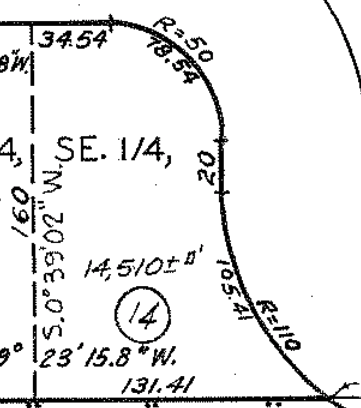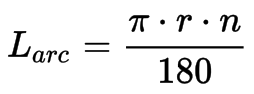# Creating a line around a certain radius circle

Tried searching this extensively here and elsewhere so pre-apologies if this is already covered.

I am working on a poor man’s version of a plot plan for an architectural drawing. It’s your basic lines set to a scale drawing. However, it’s based off off typical city engineering plans which sometimes have curves (like curves in a road). Those curves have two components: a line length and an “R” variable which stands for the diameter of a circle which would represent the arc of the curve in question. So for example you can have a line that is 75 feet long and “R=50” meaning to represent the arc in that line is the same thing you’d get off a 50’ diameter circle.

I cant find anywhere to input the value of an angle or a line relative to the scale of the document. I’ve seen talk about pixel values in two adjoining lines but that won’t get the value you’re looking for here. I saw a more recent post talk about utilising an Adjustable Arc object but that didn’t work for me in my attempt- I basically made an arc of the prescribed length, then set its points at 0 and 90, and then in the Geometry section specified a 50’ height and it didn’t seem to check out.

I feel like there has to be something basic I’m missing and hoping someone else has tried to do a line like this. I attached a picture of the exact plot I’m trying to draw where you can see the dimensions in feet (decimals) as well as the two lines and their R values in question.

Many thanks to any ideas!!Are you sure R is the diameter and not the radius of the circle? “R” means radius usually.

Yeah, as I suspected, in the above drawing R means radius, not diameter. I successfully recreated the drawing in Omnigraffle using arcs and the formula below to calculate the angles for the arcs (n being the angle).Thanks for looking into this, I really appreciate it. However in my novice talent level I can’t figure out where to input any technical information for an arc object.

What you need to input in Omnigraffle is the width/height of the adjustable arc shape (which are equal to the diameter of the whole circle) and its angle.

• You know the radius R, so the width and the height of the arc shape will both be 2*R. You can set these using the fields on the Geometry panel.
• You know the length of the arc, so the angle can be calculated using the formula I posted above. Unfortunately in Omnigraffle the start/end angles can only be set by dragging the handles of the arc, which is cumbersome.

OK, I think one of the major things I was doing wrong was setting the height and width incorrectly. I was treating width like a line length which obviously it is not since OG isn’t treating an arc like a line from what I can tell.

So if I drag an arc and set the handles to 0° and 90° and then set the height and width to 2r, in this case “100” I seem to get exactly what I need. If I build out the drawing from the original post everything seems to check out:

So I’m guessing that as long as your arc is exactly 0 to 90 and the height and width and equal maybe you dont need to calculate for n?

Thanks a lot again.